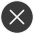Cart: 0 itemsLanguage

# PrintingFAY DREAM
RM 49.00
RM 29.00
41%VOS
RM 28.00VOS
RM 33.00Matrix MaxMatrix MaxMatrix MaxMatrix MaxMatrix MaxMatrix MaxMatrix MaxMatrix MaxMatrix MaxMatrix MaxMatrix MaxMatrix MaxMatrix MaxMatrix MaxMatrix MaxMatrix MaxMatrix MaxMatrix MaxMatrix MaxMatrix MaxMatrix MaxTOP